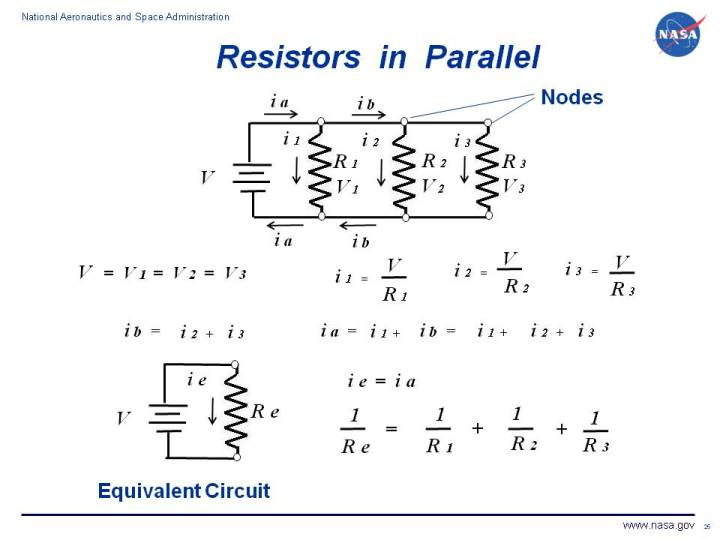+ Text Only Site
+ Non-Flash Version
+ Contact GlennElectrical circuits are used throughout aerospace engineering, from flight control systems, to cockpit instrumentation, to engine control systems, to wind tunnel instrumentation and operation. The most basic circuit involves a single resistor and a source of electric potential or voltage. Electrons flow through the circuit producing a current of electricity. The resistance, voltage, and current are related to one another by Ohm's law. There is usually more than one resistor used in a practical circuit. When analyzing a complex circuit, we can often group components together and develop an equivalent circuit. In the analysis of circuits with multiple resistors, we must determine if the resistors are subject to the some voltage or to the same current. Multiple resistors in a parallel circuit are subjected to the same voltage. Multiple resistors in a series circuit are subjected to the same current. On this page we discuss the equivalent circuit for resistors in parallel. On the figure, we show a circuit consisting of a power source and three resistors connected in parallel. If we denote resistance by R, current by i, and voltage by V, then Ohm's law states that for each resistor in the circuit: V = i R i = V / R If we consider each resistor separtely, each resistor has its own current (i1, i2, and i3), resistance (R1, R2, and R3), and voltage (V1, V2, and V3). Because the resistors are connected parallel to each other, the voltage across each resistor is the same: V = V1 = V2 = V3 The current through each resistor is determined by Ohms law: i1 = V / R1 i2 = V / R2 i3 = V / R3 If we denote the intersection of the wires connecting the resistors as nodes, there are six nodes in our three resistor circuit. On the figure, we tag the two nodes at the upper right of the circuit. At each node, the current coming into the node must equal the current leaving the node according to Faraday's Law. For the nodes located above and below resistor R2, the current ib entering the node is given by: ib = i2 + i3 Similarly for the nodes above and below resistor R1, the current ia entering the node is given by: ia = i1 + ib = i1 + i2 + i3 We now know the voltage, resistance, and current through every part of the circuit. If we were to construct an equivalent circuit as shown at the lower left, we would have the same voltage V, the same current from the power source ie = ia, and a single equivalent resistor Re. For our equivalent circuit, Ohm's law specifies that: ie = V / Re We can determine the value of Re by using a little algebra: V / Re = ie = i1 + i2 + i3 V / Re = V / R1 + V / R2 + V / R3 1 / Re = 1 / R1 + 1 / R2 + 1 / R3 1 / Re = (R2 R3 + R1 R3 + R1 R2 / (R1 R2 R3) Re = (R1 R2 R3) / (R2 R3 + R1 R3 + R1 R2) We can use this knowledge of the parallel resistor circuit to analyze the Wheatstone bridge circuit that is used for temperature control for wind tunnel force balances using electronic strain gages. Navigation ..Beginner's Guide Home Page+ Inspector General Hotline + Equal Employment Opportunity Data Posted Pursuant to the No Fear Act + Budgets, Strategic Plans and Accountability Reports + Freedom of Information Act + The President's Management Agenda + NASA Privacy Statement, Disclaimer, and Accessibility CertificationEditor: Nancy Hall NASA Official: Nancy Hall Last Updated: May 13 2021 + Contact Glenn